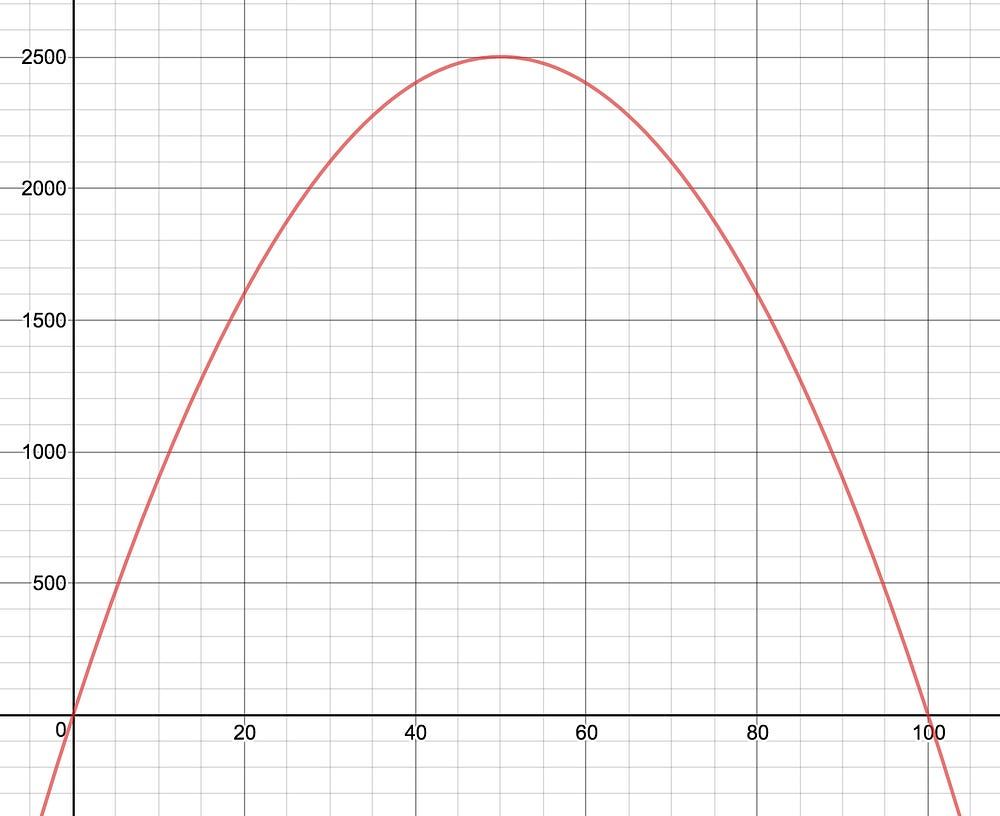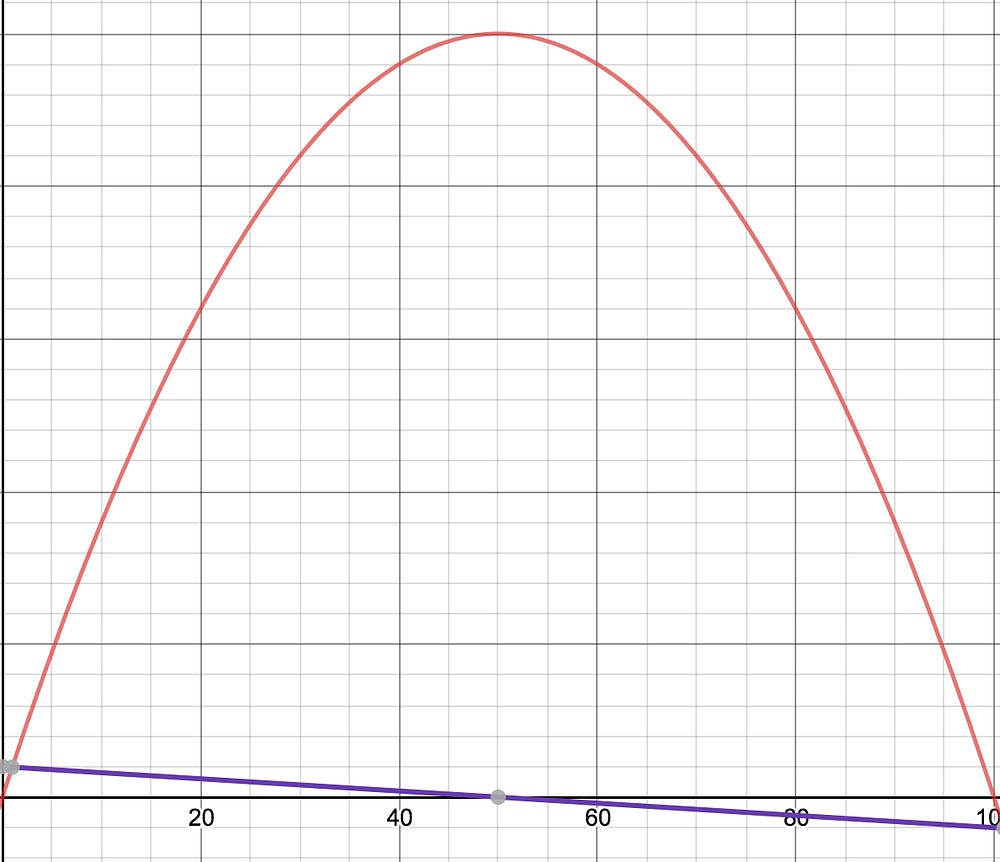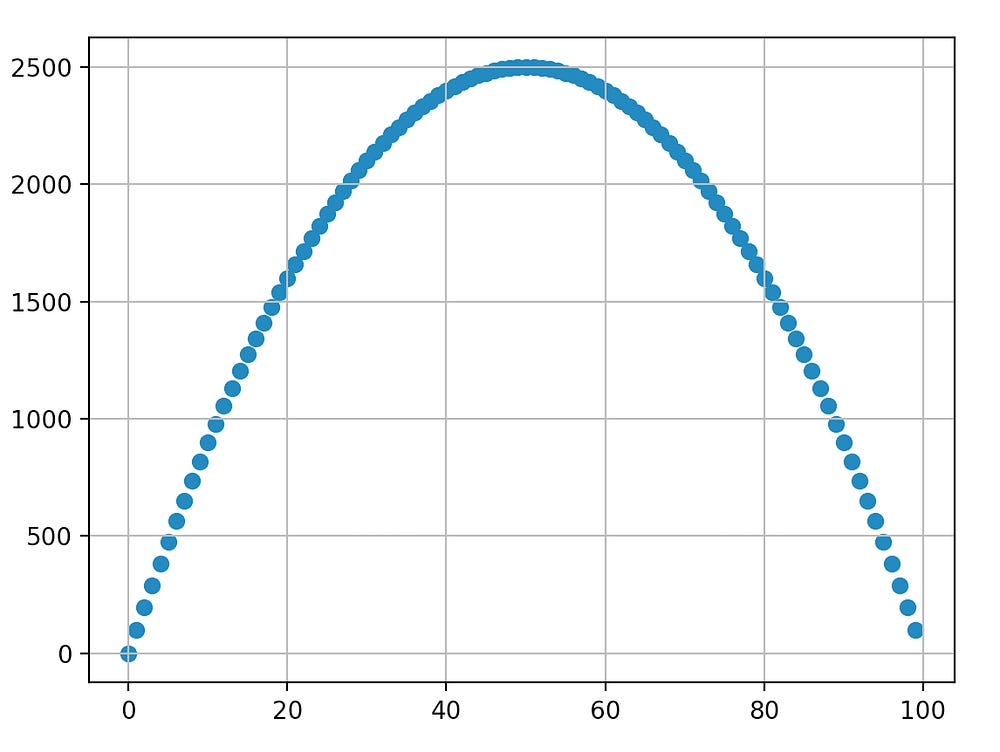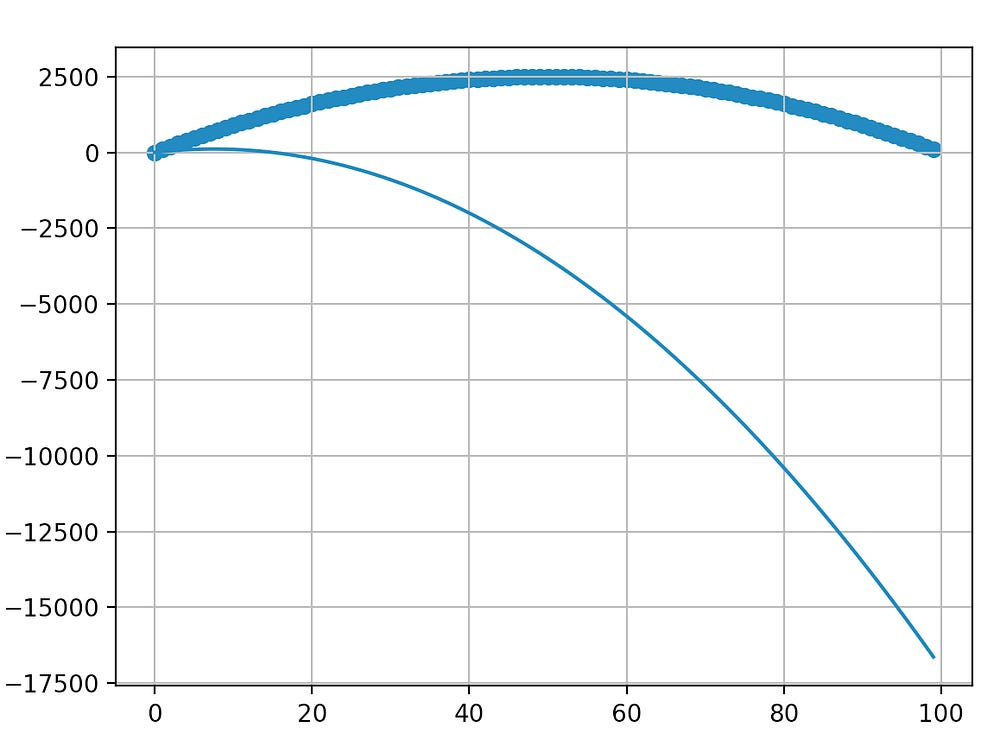# Gradient Descent

Source: Deep Learning on Medium

## Machine Learning is Applied Calculus

This is the fourth article in a series and it’s about gradient descent, a fundamental tactic for training many machine learning models including neural networks. You can find the first article in the series here, and the previous article here.

Gradient descent, and variants of it, are a widely used in machine learning as a critical part of the training process. Gradient descent is named after the multivariable generalization of the derivative, which is called the gradient.

You do not need to have heard of gradient descent before, but the rest of this article assumes that you:

• Can compute simple derivatives, partial derivatives, and the gradients of simple multivariable functions, and
• Understand the use of the derivative and gradient to find the “direction of maximum increase.”

Gradient descent is an iterative method for solving “optimization problems” — math problems revolving around finding global minimums or maximums of a function. For simple optimization problems gradient descent is not required, as we’ll soon see. We rely on iterative methods such as gradient descent when things get complex — and neural nets are sufficiently complex.

At the outset, note that the purpose of this article is not to teach you how to implement gradient descent, but to demonstrate what it is and how it is used in machine learning. At the end of this article there are links to a couple tutorials for those who want to dig deeper and implement gradient descent from scratch.

There are plenty of articles, videos, lectures, et cetera about gradient descent that rely heavily on metaphors and hand-waving about balls rolling downhill. Such an intuitive approach to describing the algorithm is definitely useful, but stopping there can leave us with the nagging feeling that we’re missing something. In an attempt to leave you without that nagging feeling that you’re missing something we will attempt to describe gradient descent concretely. First, let’s quickly review what we mean by optimization problems.

### Reviewing Optimization Problems and Calculus

You have purchased 200 meters of wire-mesh fencing. You want to use this fence to create a rectangular pasture for your flock of sheep. How can you determine the length and width that maximizes the area inside of your pasture?

Using the standard analytical approach to this problem, we would first write an equation to represent our problem. First, we know two things:

`area = length * width(2*length) + (2*width) = 200`

But we want to express area in terms of a single variable, instead of two, so we can solve the second of those two equations for width:

`2*width = 200–2*lengthwidth = [200–2*length] / 2width = 100 — length`

So, now we can substitute 100 — length for width:

`A(length) = length * [100 — length]A(length) = 100*length — length²`

The next step is to take the derivative of A(length):

`A’(length) = 100–2*length`

Now, we find the “critical points,” of our function — the values for length where the first derivative is equal to zero and therefore the slope of our function at those points is also zero. We care about these values of length, because they are the only ones that could be minimums or maximums. At a critical point, it’s possible for the values on either side of that point to both be smaller or both be larger than the value at the critical point — everywhere else in the function the slope is non-zero, which means the value on one side well be less than the value at length, and the value on the other side will be more than the value at length — that is, the function is either increasing or decreasing at non-critical points and therefore cannot be a maximum or minimum point.

`A’(length) = 0A’(length) = 100–2*length`
`100–2*length = 0-2*length = -100length = 50`

This tells us that, if there is a global maximum or minimum, it must occur at length = 50. Which is to say, if there is an optimum choice for the length of our rectangle then it’s 50 feet. Because it’s possible to have critical points that are neither a minimum or maximum we should test that the critical point we found was indeed an extrema and not a shoulder. In calculus class you might have learned to use the “second derivative test” for this, but let’s do something a little simpler (and more like gradient descent) — We’ll just test 2 points on either side of the critical point length = 50.

`A(length) = 100*length — length²a(49) = 4900–2401 = 2499a(50) = 5000–2500 = 2500a(51) = 5100–2601 = 2499`

This isn’t really proof that some point in between 49 and 50 or 50 and 51 isn’t better than 50 — but this is the kind of approximation we will ultimately use in the process of machine learning. For this problem we might have had an easier time just graphing the function:A global maximum appears at length=50 — just like the derivative told us.

### Gradient Descent — Iterative Guesswork

So, what does all this have to do with gradient descent? Gradient descent is brutish, like the imprecise guesswork we just did. It’s not an elegant formula, it’s a messy series of ever so slightly better guesses. Here’s how it would work for our fencing problem:

1. Make a guess about the optimum length of the fence.
2. Compute the value of the derivative at that point.
3. Based on the value of the derivative, adjust your guess.
4. Repeat until you guess right.

Let’s say we randomly decided to guess 57 as the first value of length. The derivative of the area function at 57 is:

`A’(57) = 100–2*(57)A’(57) = -14`

The slope at length=57 is not 0, so it’s not a critical point. Furthermore, the slope being -14 suggests that if we increase length by 1 then A(length) will decrease by 14 (assuming the slope of the function doesn’t change). Gradient descent uses this value as a guide for making our next guess — we want to increase the value of A(length). Because the slope was negative we should decrease or next guess for length in order to increase the value of A(length).

There is no a priori way of knowing exactly the “right” amount to adjust our guess, even though we know the direction. In a package like Keras or TensorFlow the amount by which we adjust our guess will be governed by a hyperparameter called the “learning rate” which you can choose at training time. Increasing the learning rate will cause gradient descent to take bigger steps; decreasing it will cause gradient descent to take smaller steps. For now, let’s say we reduce our guess by 3 — it’s kind of arbitrary but that’s actually okay.

`57–3 = 54A(54) = 5400 — (54²)A(54) = 5400–2916A(54) = 2484`

2484 is bigger than 2451, we call that progress. Once again we use the derivative to check if we’re at a critical point and adjust our guess if we’re not:

`A’(length) = 100–2*54 = 100–108 = -8`

We still guessed too high and 8 is less than 14 so lets reduce our guess less — why not by 2?

`A(52) = 5200–52²A(52) = 5200–2704A(52) = 2496`

And we’d repeat this process until we found a critical point, or until the value of the derivative was so close to zero that we call it close enough to a critical point. This process is repetitive and tedious to do by hand, but computers are fantastic at repetitive and tedious arithmetic. This is also where the hill climbing and rolling ball metaphors come into play — we just keep going up the parabola until we can’t go up any further!Notice the derivative (blue) — it’s negative to the right of the maximum, and positive to the left of the maximum. In this case it’s easy to follow the derivative directly the the max.

This example would be better named gradient ascent since we were trying to find a maximum value. In gradient descent we just negate the value of the gradient each time we compute it. Otherwise it’s this same process: guess until you find a critical point, then stop and evaluate.

There are two big differences between how we applied gradient descent just now, and how it’s used in neural networks. First, the neural network represents a function that is much more complex than A(length) = 100*length — length². Our neural networks have hundreds of thousands of tunable parameters, where we just had one: length. The massive complexity of neural networks is the primary reason we an iterative process like gradient descent, rather than the analytical process we first used to find all the critical points and evaluate them — computing the general form of the derivative of the function our neural network represents is simply not feasible at the scale of most neural nets.

The second difference is that in this example we had a real function that served as ground truth, and we found the optimum value of that known function. In neural networks there is no “real” function that’s being optimized; instead we are trying to create a function where no ground truth function exists, only whatever data points we have to train the network. When we use a deep neural network, we’re asserting a function exists that approximates our data — and we’re trying to find that a function.

### Gradient Descent For Function Finding

In the prior example we had a curve and we used gradient descent to find an optimal value along that curve. In machine learning we have a collection of data points and we want to create a curve that satisfactorily fits those data-points. Let’s take the fencing problem we just examined and make it more like the problems we tend to solve with machine learning:

We have a collection of data points from a fence building database. Each data point in our dataset is drawn from rectangular pastures that were built with exactly 100 meters of fencing material. Each data point has 2 parameters: the length of one side of the fence, and the area of the fence. In this version of the problem we’re not trying to find the optimum length of our fence — we’re trying to find a function that can predict the area of a rectangular fence given the length of one side. We happen to know that function already… it’s A(x) = 100x * x², but our machine learning system doesn’t know that yet. Our input data would look something like this:It looks like there is a pattern in this data… can we use machine learning to define the pattern?

The machine learning approach to this problem is to think, “gee, that looks kind of like some kind of mathematical function… but I wonder which function exactly?” Let’s take it a step further and say we smartly guessed that this function was some kind of parabolic function. We write down a template for the function that (we hope) will fit this data:

`F(x) = ax² + bx`

This function will be correct when a = -1 and b=100. We already have all of the x and y values in the dataset, now we’re going to use gradient descent to find the best values of a and b. We do this by introducing yet another function called the loss function, and running gradient descent to minimize the loss function. Let’s use F(x) as “the function being trained”, where x is still the length of one side of the pasture, y is the true (observed) area of that pasture. The absolute error of a single prediction is:

`L(x) = | F(x) — y |`

We have to take the absolute value of the error. If we don’t then making two guesses where one is off by 1000 and the other is off by -1000 would be the same as being correct even though we were actually very wrong twice in a row.

Let’s choose a simple loss function: the mean absolute error, which is just the average of the above L(x) over all the points in our dataset. It’s more common to use a metric like mean squared error, but different loss functions will apply better to different datasets. For now let’s continue using the mean absolute error as our loss function.

Suppose we randomly selected starting values for our weights: a=-2 and b=30. Expanding F(x) the loss function over a single point becomes:

`L(x) = | -2x² + 30x + 0 — y |`

If we plot our template function next to our data points, we can see that the error will vary from point to point, and that there are lots of errors so sum up.Oops, we start pretty close to our scatterplot points at x=0 but by the time x=100 we are way off. The absolute error of a single datapoint the length of the vertical line that connects a point in the scatter plot to the point below it on the line. The mean absolute error is the average length of all those vertical lines.

This next part gets tricky. We want to adjust the values of a and b in order to minimize the loss function. So we have to compute the derivative of the loss function with respect to a, b, and c, thats 3 variables so we need the gradient. Previously, we had been writing the function as though x was the value that changed — but in this version of the problem, that’s not the case. The value for x is always just some fixed value from our dataset. So let’s rewrite the loss function for a single data point:

`L(a, b) = ax² + bx — y; where x and y are treated as constants.`

And the true loss function, the mean absolute error:

`L(a, b) = 1/m * SUM(| F(a, b) — yi | )L(a, b) = (1/m) * SUM( | axi² + bxi — yi | )`

Where xi and yi represent a single datapoint from our dataset and m is the number of points in our dataset. There are two subtle problems here — one is that we have to use the chain rule to compute a derivative involving the absolute value. The other is that the absolute value function has a corner and is not differentiable at the point where our prediction are exactly equal to the true values. Lucky for us the derivative of the absolute value is easy:

`d/dx |x| =  1 if x is positive andd/dx |x| = -1 if x is negative.`

We can solve the corner problem by stopping gradient descent if the mean absolute error was 0. This makes practical sense — if we guessed exactly right on every single data point then it’s no longer possible to adjust the model in a way that improves the absolute error, because the error was 0.

So, let’s take the two partial derivatives that make up the gradient.

`L(a, b) = (1/m) * SUM( | axi² + bxi — yi | )`
`L’a(a, b) = (1 / m) * SUM( 1 * xi² );  if F(xi) > yiL’a(a, b) = (1 / m) * SUM( -1 * xi² ); if F(xi) < yi`
`L’b(a, b) = (1 / m) * SUM( 1 * x );  if F(xi) > yL’b(a, b) = (1 / m) * SUM( -1 * x ); if F(xi) < yi`

These derivatives actually give us a pretty simple update rule: if our predictions are too small then make a and b both bigger. If our predictions are too big then make a and b both smaller. Here is a short python script that will quantify the error of our chosen a and b. Recall a=2 and b=30

`import numpy as np`
`x = np.arange(0,100,1)y_true = [100*p — p*p for p in x] # b = 100 a = 1, the ground truthy_est = [30*p — 2*p*p for p in x] # b = 30 a = 2, our guess`
`error = sum([ye — yt for ye, yt in zip(y_est, y_true)])mean_error = error / 100`
`# error = -674850# mean_error = -67485`

Our predictions result in a negative raw error value, meaning F(x) < y. So we use the bottom form of both of the partial derivatives. Our direction of maximum increase is negative in both the a and b directions — the direction of maximum decrease then is positive in both the a and b directions. Which we know is right, we guessed a = -2, and the true a = -1; we guessed b=30 and the true b=100, in both cases we guessed too low.

These partial gradients are also telling us that the weight on x² (the value we’re calling a) is likely more responsible for the error we’re experiencing. This might be slightly confusing, because our guess for a was only off by one, but we were off by 70 in our guess for b. It’s not wise to put too much meaning in the exact values of the gradients in the a and b directions. The gradient is just telling us that the a direction has more power to contribute to error — being off by a factor of 50 on the x² term will create more error than being off by 50 on the x term. We want to update our weights a and b according to the gradient, we should move both of them a little bit in the positive direction.

Let’s say we do that, and end up with a = -.5 and b = 33. If we graph that it looks like this:

The absolute error is significantly better with these values for a and b. Just like before, we can continue to slowly adjust our guesses for a and b until our absolute error is acceptably small.

Lots can go wrong during gradient descent. Your descent might thrash around by changing the guesses by too much at each step, bouncing back and forth between wrong guesses. It might not update the values by enough, which would cause it to converge on a critical point very slowly. In functions with multiple critical points, gradient descent might get stuck in a local minimum or at a saddle point instead of finding a global minimum.

Luckily, modern machine learning packages ship with gradient descent built in, meaning practitioners don’t have to develop gradient descent from scratch. Not only that, but a ton of of work has gone into extending the idea of gradient descent into other optimizers, that are more efficient at finding maxima and minima. Nevertheless, understanding gradient descent and the idea of optimizing a loss function at a high level can help practitioners think about problems like overfitting. It also helps them better understand the process of training a neural network.

If you want to dig deeper, consider attempting these tutorials about implementing gradient descent from scratch. Doing so will help you become familiar with some of the trickiest edge cases and implementation details (but, honestly, not doing it won’t hinder your ability to be an effective machine learning practitioner — dealers choice):

In a future article, we’ll explore backpropagation which was the original breakthrough that allowed computer scientists to apply gradient descent to neural networks. The short version is: backpropagation is a clever way to efficiently compute the partial gradient for each of the thousands of weights in the computational graph that is our neural network. See you then!

This article was produced by Teb’s Lab. To learn more about the latest in technology sign up for the Weekly Lab Report, become a patron on Patreon, visit our website, or just follow us here on Medium.